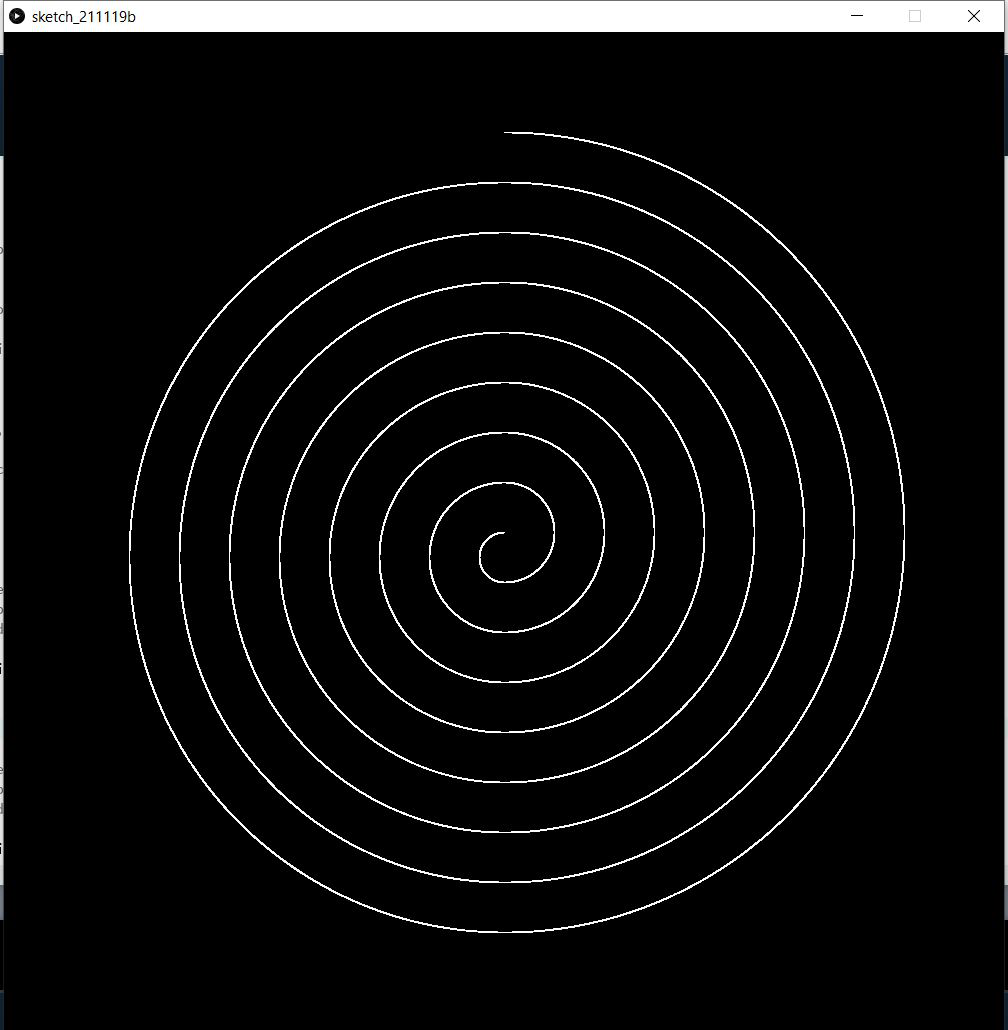Open in App
Not now

# How to draw a Spiral using arcs in Processing with Python Mode?

• Last Updated : 19 Dec, 2021

Processing is a programming language, and a development environment. It is an open-source software to create visual arts, graphics and animation using programming. It supports around 8 different modes, and in this article, we will be using Python Mode.

In this article, we are going to draw a spiral made of arcs using Processing with Python Mode. In case if you have not installed the Processing Software yet, follow this How to setup Python mode for Processing to download and install Processing and setup Python Mode. Here is a preview of what we are going to do. We are going to draw this spiral.### Approach:

• Firstly open Processing software and choose Python Mode.
• Then in the coding part, we are going to define two functions, setup() and draw().
• setup() function is called before the start of the program. Here we have to set the background color and size of the window.
• In the draw() function, we are first setting the fill color of arcs to None using noFill() function. Then stroke color of arcs to white color.
• After that, we are using a loop to create arcs using arc() function. In each iteration we have to increase the height and width of the arc by 50px and tilt the arc by 180 degrees, to form a spiral.

Below is the full implementation:

Here is the Python code for the above approach. Now type the following code in the code editor.

## Python3

 `# function to setup size of ` `# output window ` `def` `setup(): ` `    ``# to set background color of window ` `    ``# to black color ` `    ``background(``0``) ` `     `  `    ``# to set width and height of window ` `    ``# to 1000px and 1000px respectively ` `    ``size(``1000``, ``1000``) ` ` `  `# function to draw on the window ` `def` `draw(): ` `   `  `    ``# to set the fill color of the arcs to None ` `    ``noFill() ` `     `  `    ``# to set the border color of arcs to white ` `    ``#that is rgb(255,255,255) ` `    ``stroke(``255``, ``255``, ``255``) ` `     `  `    ``# loop to create 16 arcs ` `    ``for` `i ``in` `range``(``16``): ` `        ``# if i is even ` `        ``if` `i ``%` `2` `=``=` `0``: ` `           `  `          ``# function to create an arc with center (500,525) ` `          ``# and width and height as 50px and 50px respectively ` `          ``# In each alternate iteration, the height and width of ` `          ``# the arcs increases by 100px. The arc starts at ` `          ``# 90 degrees that is half times PI and end at 270 ` `          ``# degrees (90 + 180) that is PI + half times PI ` `            ``arc(``500``, ``525``, ``50``+``i``*``50``, ``50``+``i``*``50``, ` `                ``HALF_PI, HALF_PI``+``PI) ` `             `  `        ``# if i is odd ` `        ``else``: ` `          ``# function to create an arc with center (500,500) ` `          ``# and width and height as 50px and 50px respectively ` `          ``# In each alternate iteration, the height and width of ` `          ``# the arcs increases by 100px. The arc starts at ` `          ``# 270 degrees that is PI + half times PI and end at 450 ` `          ``# degrees (90 + 360) that is 2 times PI + half times PI ` `            ``arc(``500``, ``500``, ``50``+``i``*``50``, ``50``+``i``*``50``,  ` `                ``HALF_PI``+``PI, HALF_PI ``+` `2``*``PI) `

Output:My Personal Notes arrow_drop_up
Related Articles